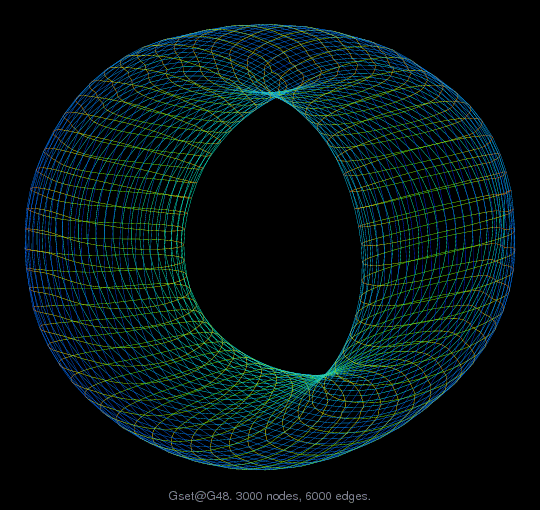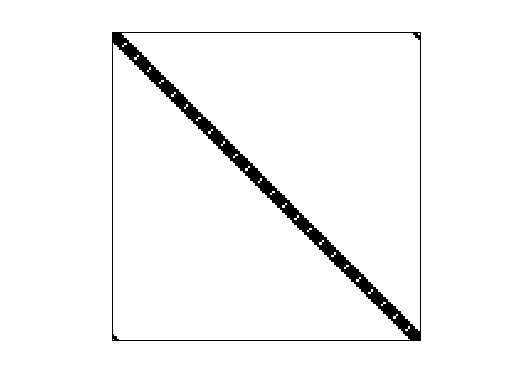Matrix: Gset/G48

Description: 2D torus, 60-by-50(undirected graph drawing)• Matrix group: Gset
• download as a MATLAB mat-file, file size: 19 KB. Use UFget(511) or UFget('Gset/G48') in MATLAB.

 Matrix properties number of rows 3,000 number of columns 3,000 nonzeros 12,000 # strongly connected comp. 1 explicit zero entries 0 nonzero pattern symmetry symmetric numeric value symmetry symmetric type binary structure symmetric Cholesky candidate? no positive definite? no

 author C. Helmberg editor Y. Ye date 1996 kind undirected random graph 2D/3D problem? no

 SVD-based statistics: norm(A) 4 min(svd(A)) 1.52374e-17 cond(A) 2.62511e+17 rank(A) 2,982 null space dimension 18 full numerical rank? no singular value gap 6.1694e+12

 singular values (MAT file): click here SVD method used: s = svd (full (A)) ; status: ok